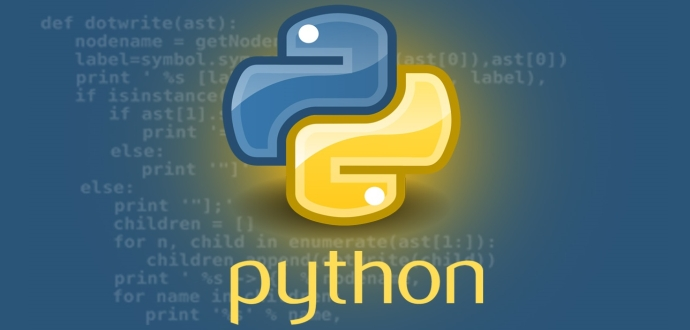# Variables

What are variables?

In programming, a variable is a named storage location that holds a value. The value can be of different types, such as numbers, text, or boolean values. The name of the variable is used to reference the value stored in that location.

Variables are useful in programming because they allow you to store and manipulate data in your programs. For example, you can use a variable to store a user's name or age, perform calculations, or keep track of program state.

Each data type has its own characteristics and uses, and we'll cover each one in more detail in the following topics.

In Python, you create a variable by using the assignment operator (=). For example, the following code creates a variable called 'x' and assigns it the value '5':

x = 5

After this statement is executed, the value '5' is stored in the variable 'x'. You can then use the variable x in other parts of your program.

How to create a variable in Python (using the assignment operator)

In Python, you create a variable by using the assignment operator (=). The general syntax for creating a variable is:

variable_name = value

Here, 'variable_name' is the name you choose for your variable, and 'value' is the value you want to assign to the variable. For example, you can create a variable called 'name' and assign it the value "Alice" like this:

Each data type has its own characteristics and uses, and we'll cover each one in more detail in the following topics.

name = "Alice"

After this statement is executed, the value "Alice" is stored in the variable 'name'.

In Python, variables are dynamically typed, which means that the data type of a variable is determined at runtime based on the value it holds. For example, you can create a variable called 'age' and assign it an integer value like this:

age = 25

Later in your program, you can change the value of a variable by assigning it a new value. For example, you can update the value of the 'age' variable like this:

age = 26

Now, the value of the 'age' variable is '26'.

Naming rules and conventions for variables in Python

In Python, there are some rules and conventions you should follow when naming variables:

• Variable names must start with a letter or an underscore (_), but not with a number or a special character.
• Variable names can contain letters, numbers, and underscores, but no other characters (e.g. spaces or punctuation).
• Variable names are case-sensitive, so 'age', 'Age', and 'AGE' are considered different variables.
• Variable names should be descriptive and give a clear idea of what the variable represents.
Here are some examples of valid and invalid variable names in Python:

# Valid variable names
name = "Alice"
age = 25
_average = 7.5
total_cost = 42.0
# Invalid variable names
# no spaces or punctuation allowed in variable names
total.cost = 42.0 # variable names are case-sensitive,
#so Total_Cost and total_cost are different variables
Total_Cost = 50.0

It's important to follow naming conventions for variables in order to write clear and readable code that other programmers can easily understand.

Examples of using variables in Python programs

Variables are used in Python programs to store and manipulate data. Here are some examples of how you can use variables in your programs

Example 1: Storing user input

You can use a variable to store user input and then use that value later in your program. For example, the following code prompts the user to enter their name, stores the input in a variable called name, and then uses the variable to print a personalized message:

name = input("What is your name? ")
print("Hello, " + name + "! Welcome to my program.")

#### BHAGAT CYBERWALA

Welcome to Web2hack! We are a group of passionate coders and cyber security experts who are excited to share our knowledge and experience with the world.